Скачать презентацию Math 307 Spring 2003 Hentzel Time 1 10

395ef748843848b6672347710747a012.ppt

• Количество слайдов: 50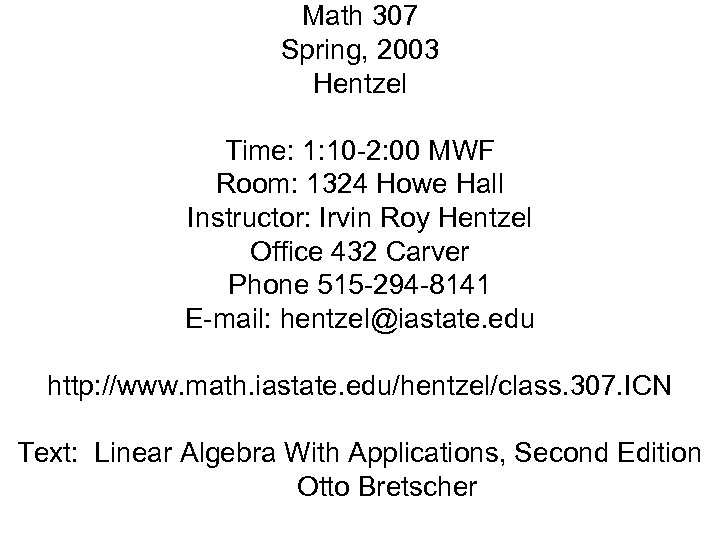Math 307 Spring, 2003 Hentzel Time: 1: 10 -2: 00 MWF Room: 1324 Howe Hall Instructor: Irvin Roy Hentzel Office 432 Carver Phone 515 -294 -8141 E-mail: [email protected] edu http: //www. math. iastate. edu/hentzel/class. 307. ICN Text: Linear Algebra With Applications, Second Edition Otto Bretscher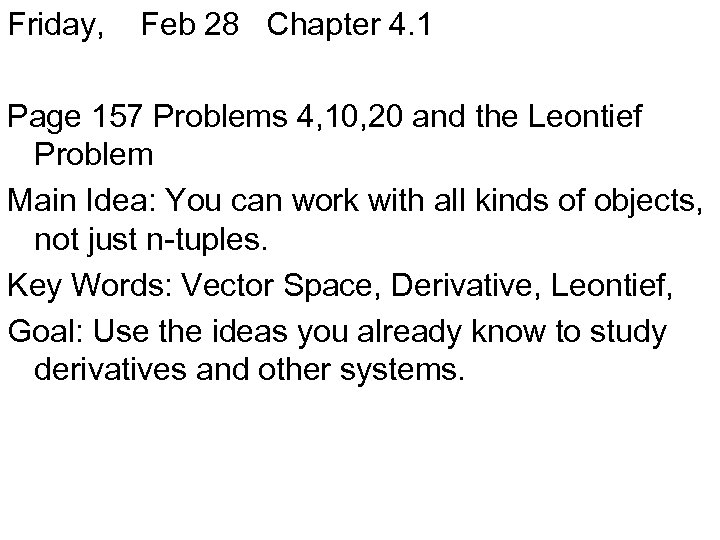Friday, Feb 28 Chapter 4. 1 Page 157 Problems 4, 10, 20 and the Leontief Problem Main Idea: You can work with all kinds of objects, not just n-tuples. Key Words: Vector Space, Derivative, Leontief, Goal: Use the ideas you already know to study derivatives and other systems.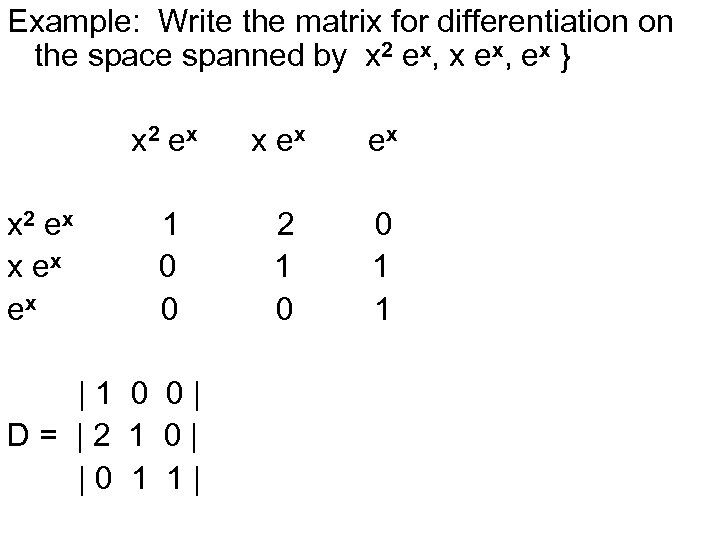Example: Write the matrix for differentiation on the space spanned by x 2 ex, x ex, ex } x 2 ex x ex ex 1 0 0 2 1 0 0 1 1 |1 0 0| D= |2 1 0| |0 1 1|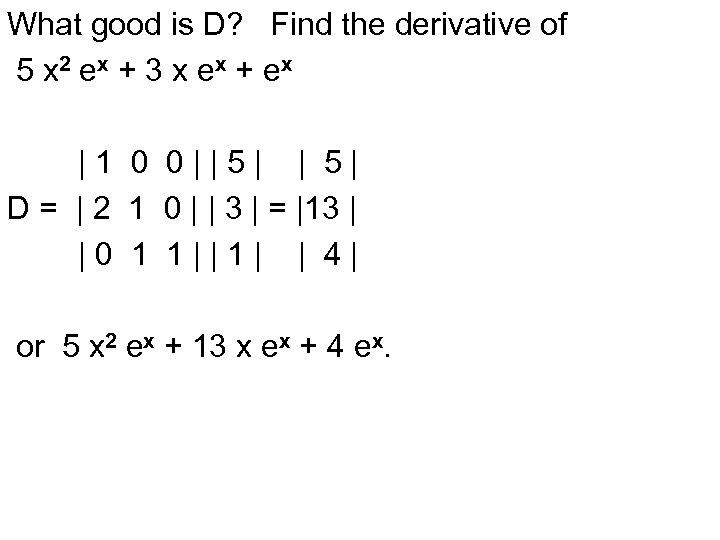What good is D? Find the derivative of 5 x 2 ex + 3 x e x + e x |1 0 0||5| | 5| D = | 2 1 0 | | 3 | = |13 | |0 1 1||1| | 4| or 5 x 2 ex + 13 x ex + 4 ex.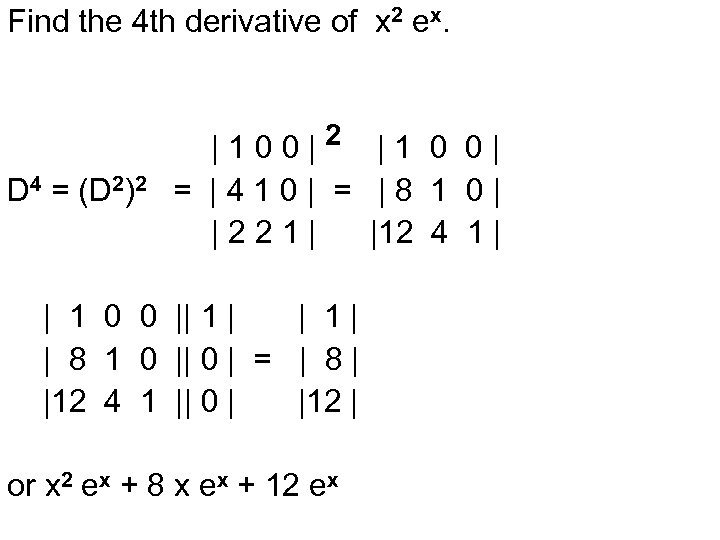Find the 4 th derivative of x 2 ex. D 4 = (D 2)2 |100|2 |1 0 0| = |410| = |8 1 0| |221| |12 4 1 | | 1 0 0 || 1 | | 1| | 8 1 0 || 0 | = | 8 | |12 4 1 || 0 | |12 | or x 2 ex + 8 x ex + 12 ex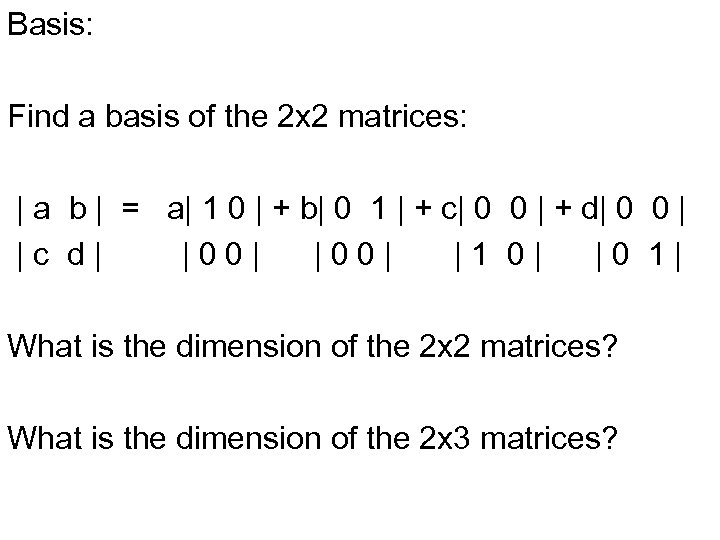Basis: Find a basis of the 2 x 2 matrices: | a b | = a| 1 0 | + b| 0 1 | + c| 0 0 | + d| 0 0 | |c d| |00| |1 0| |0 1| What is the dimension of the 2 x 2 matrices? What is the dimension of the 2 x 3 matrices?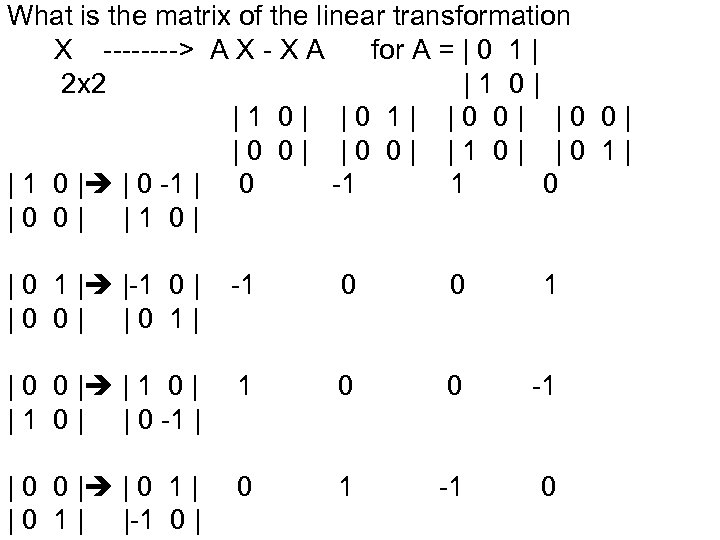What is the matrix of the linear transformation X ----> A X - X A for A = | 0 1 | 2 x 2 |1 0| |0 1| |0 0| |1 0| |0 1| | 1 0 | | 0 -1 1 0 |0 0| |1 0| | 0 1 | |-1 0 | |0 0| |0 1| -1 0 0 1 | 0 0 | | 1 0 | | 0 -1 | 1 0 0 -1 | 0 0 | | 0 1 | |-1 0 | 0 1 -1 0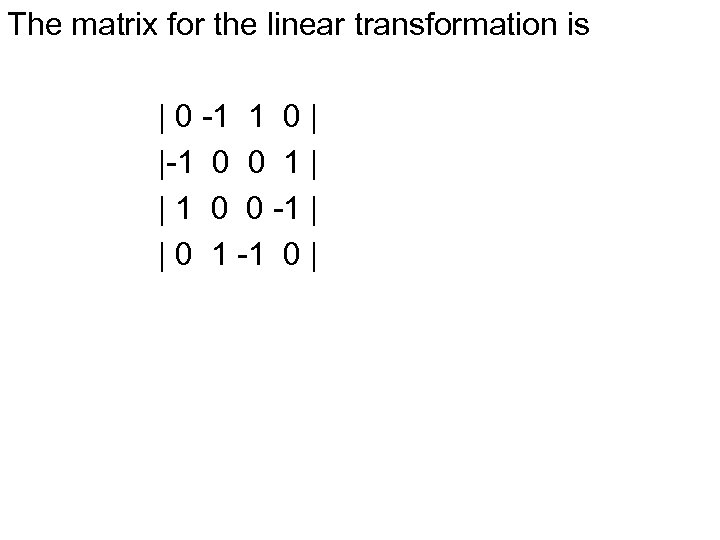The matrix for the linear transformation is | 0 -1 1 0 | |-1 0 0 1 | | 1 0 0 -1 | | 0 1 -1 0 |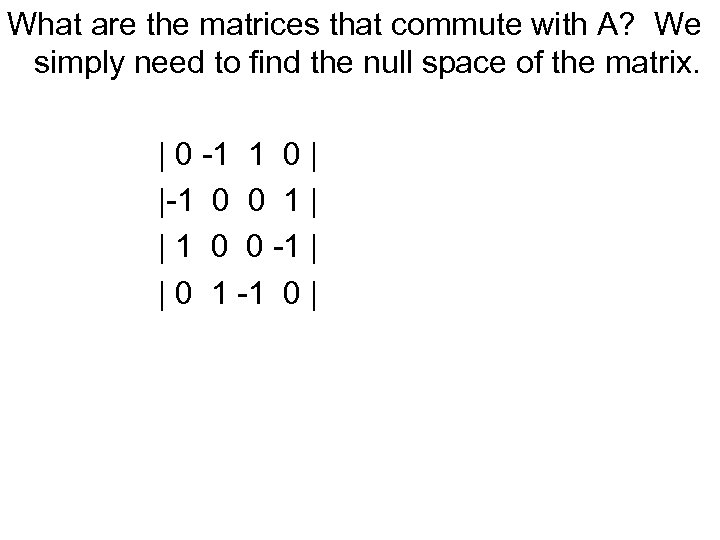What are the matrices that commute with A? We simply need to find the null space of the matrix. | 0 -1 1 0 | |-1 0 0 1 | | 1 0 0 -1 | | 0 1 -1 0 |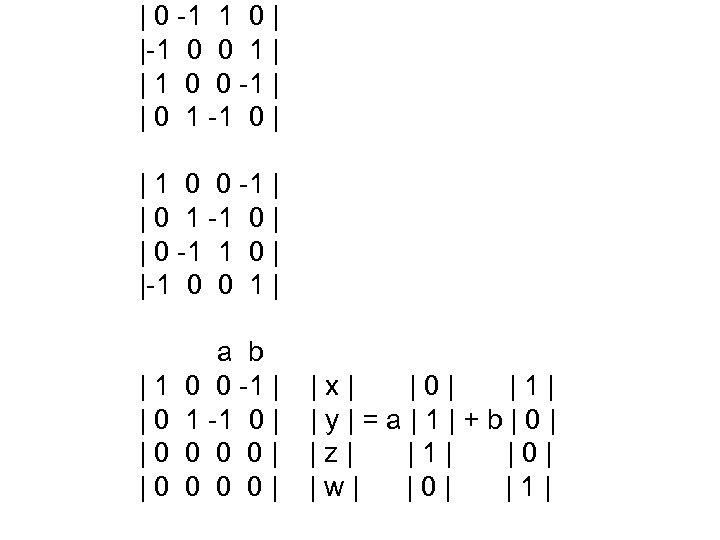| 0 -1 1 0 | |-1 0 0 1 | | 1 0 0 -1 | | 0 1 -1 0 | | 0 -1 1 0 | |-1 0 0 1 | |1 |0 |0 |0 a b 0 0 -1 | 1 -1 0 | 0 0 0| |x| |0| |1| |y|=a|1|+b|0| |z| |1| |0| |w| |0| |1|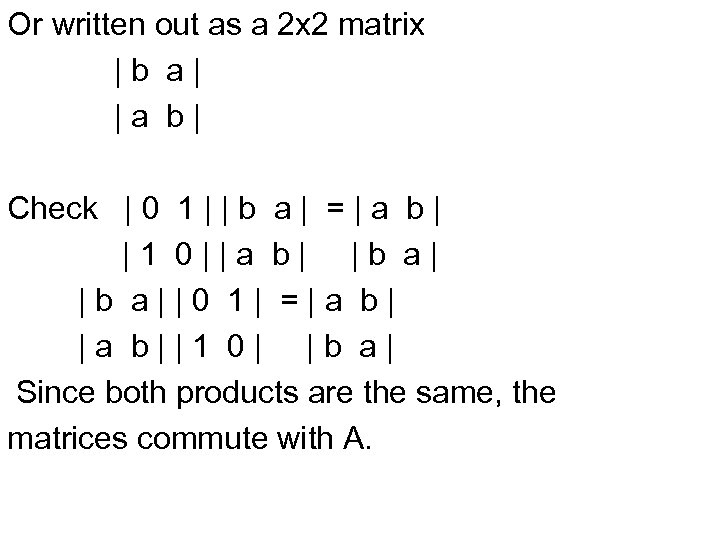Or written out as a 2 x 2 matrix |b a| |a b| Check | 0 1 | | b a | = | a b | |1 0||a b| |b a||0 1| =|a b||1 0| |b a| Since both products are the same, the matrices commute with A.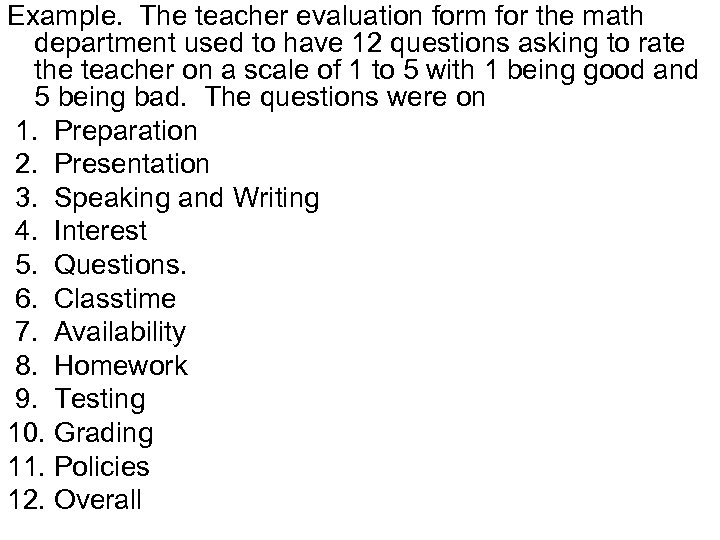Example. The teacher evaluation form for the math department used to have 12 questions asking to rate the teacher on a scale of 1 to 5 with 1 being good and 5 being bad. The questions were on 1. Preparation 2. Presentation 3. Speaking and Writing 4. Interest 5. Questions. 6. Classtime 7. Availability 8. Homework 9. Testing 10. Grading 11. Policies 12. Overall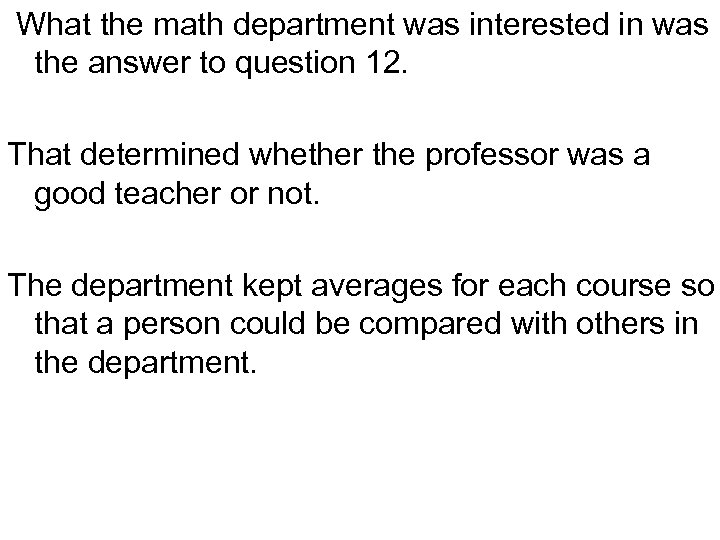What the math department was interested in was the answer to question 12. That determined whether the professor was a good teacher or not. The department kept averages for each course so that a person could be compared with others in the department.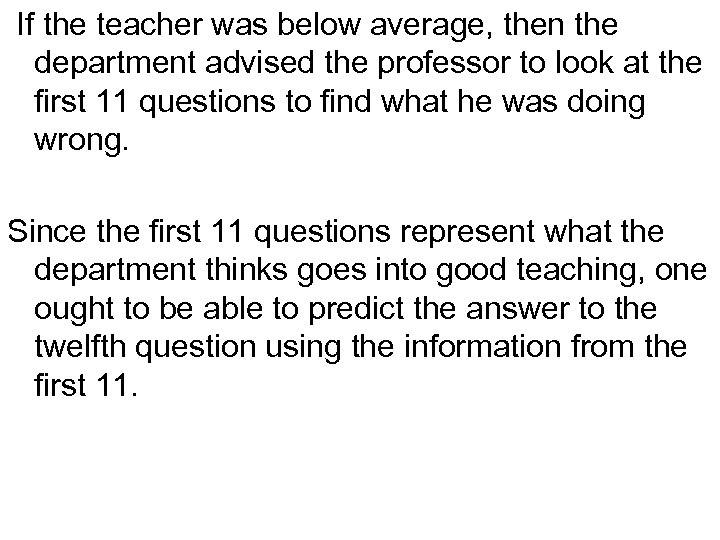If the teacher was below average, then the department advised the professor to look at the first 11 questions to find what he was doing wrong. Since the first 11 questions represent what the department thinks goes into good teaching, one ought to be able to predict the answer to the twelfth question using the information from the first 11.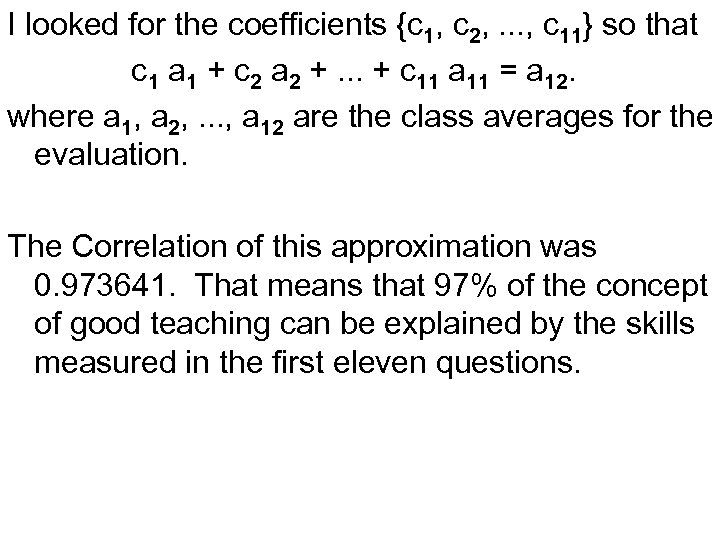I looked for the coefficients {c 1, c 2, . . . , c 11} so that c 1 a 1 + c 2 a 2 +. . . + c 11 a 11 = a 12. where a 1, a 2, . . . , a 12 are the class averages for the evaluation. The Correlation of this approximation was 0. 973641. That means that 97% of the concept of good teaching can be explained by the skills measured in the first eleven questions.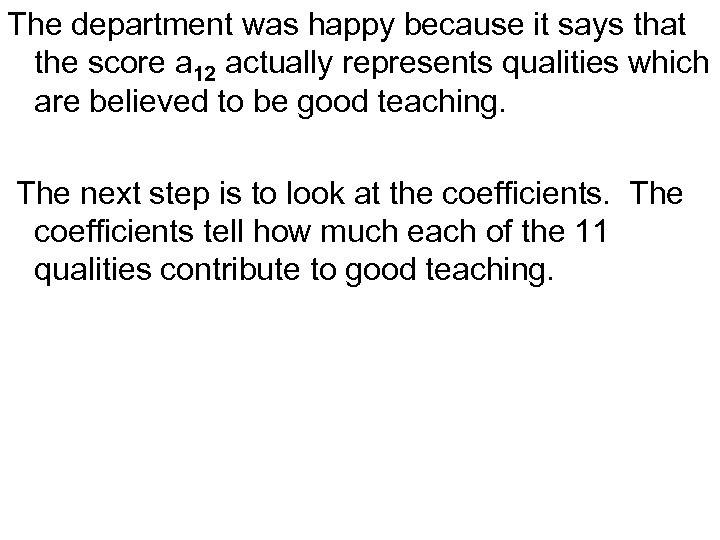The department was happy because it says that the score a 12 actually represents qualities which are believed to be good teaching. The next step is to look at the coefficients. The coefficients tell how much each of the 11 qualities contribute to good teaching.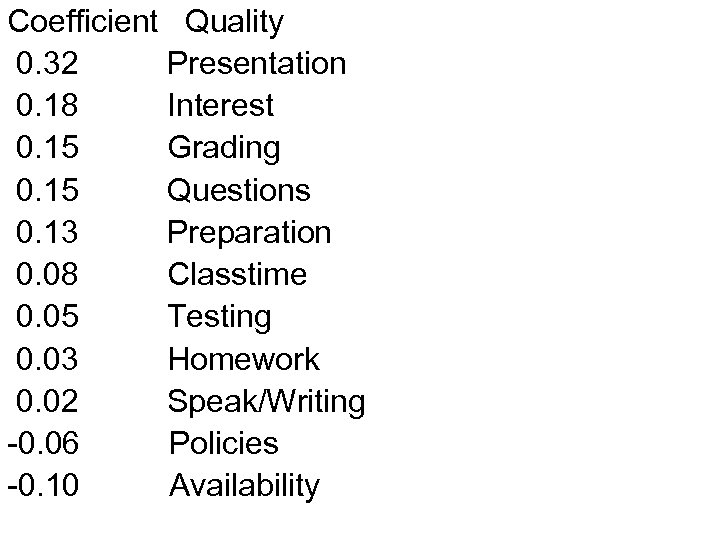Coefficient Quality 0. 32 Presentation 0. 18 Interest 0. 15 Grading 0. 15 Questions 0. 13 Preparation 0. 08 Classtime 0. 05 Testing 0. 03 Homework 0. 02 Speak/Writing -0. 06 Policies -0. 10 Availability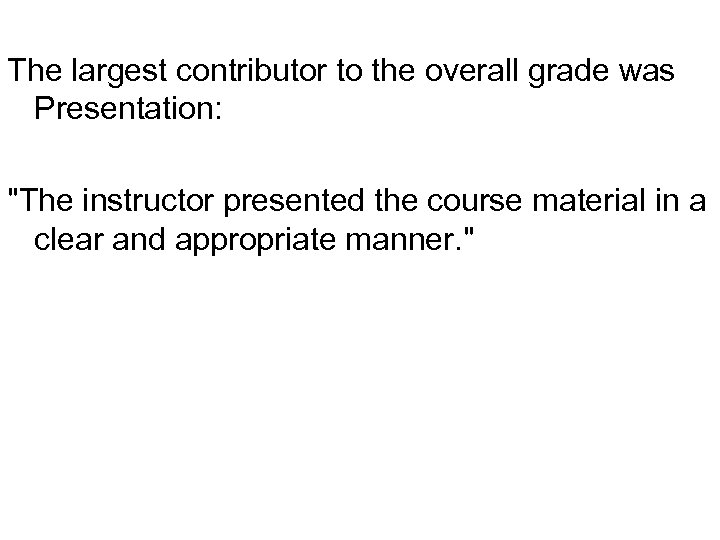The largest contributor to the overall grade was Presentation: "The instructor presented the course material in a clear and appropriate manner. "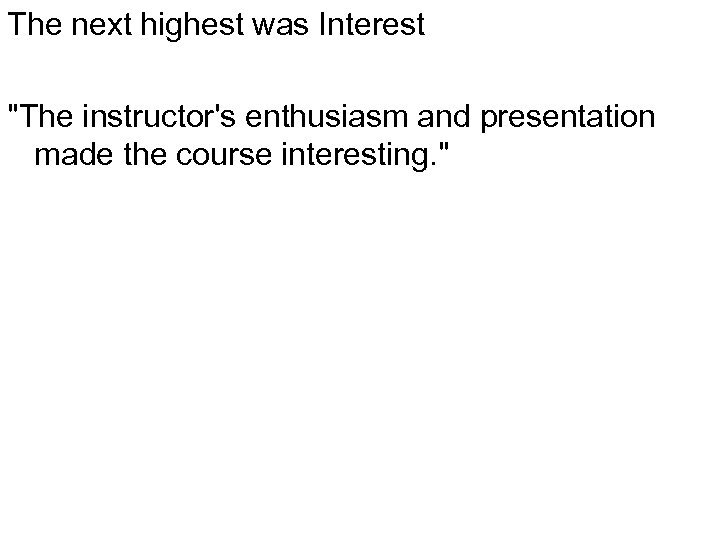The next highest was Interest "The instructor's enthusiasm and presentation made the course interesting. "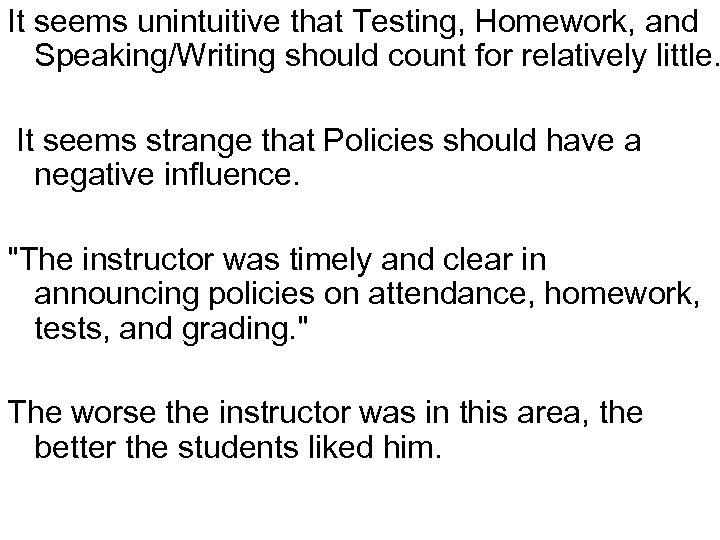It seems unintuitive that Testing, Homework, and Speaking/Writing should count for relatively little. It seems strange that Policies should have a negative influence. "The instructor was timely and clear in announcing policies on attendance, homework, tests, and grading. " The worse the instructor was in this area, the better the students liked him.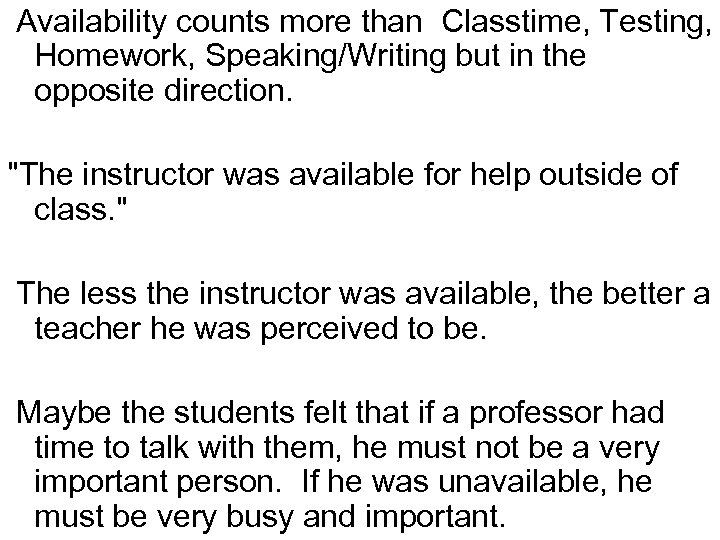Availability counts more than Classtime, Testing, Homework, Speaking/Writing but in the opposite direction. "The instructor was available for help outside of class. " The less the instructor was available, the better a teacher he was perceived to be. Maybe the students felt that if a professor had time to talk with them, he must not be a very important person. If he was unavailable, he must be very busy and important.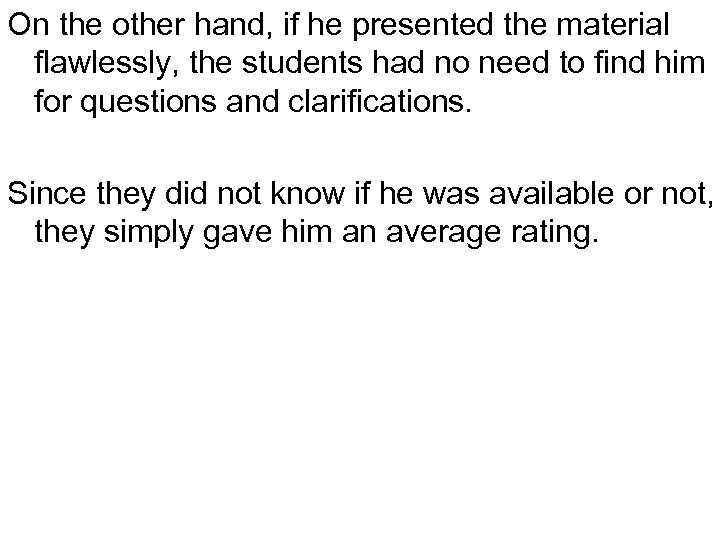On the other hand, if he presented the material flawlessly, the students had no need to find him for questions and clarifications. Since they did not know if he was available or not, they simply gave him an average rating.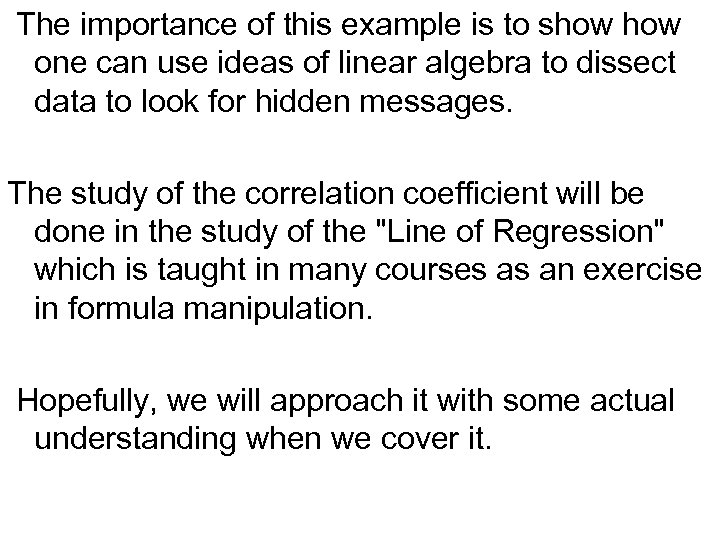The importance of this example is to show one can use ideas of linear algebra to dissect data to look for hidden messages. The study of the correlation coefficient will be done in the study of the "Line of Regression" which is taught in many courses as an exercise in formula manipulation. Hopefully, we will approach it with some actual understanding when we cover it.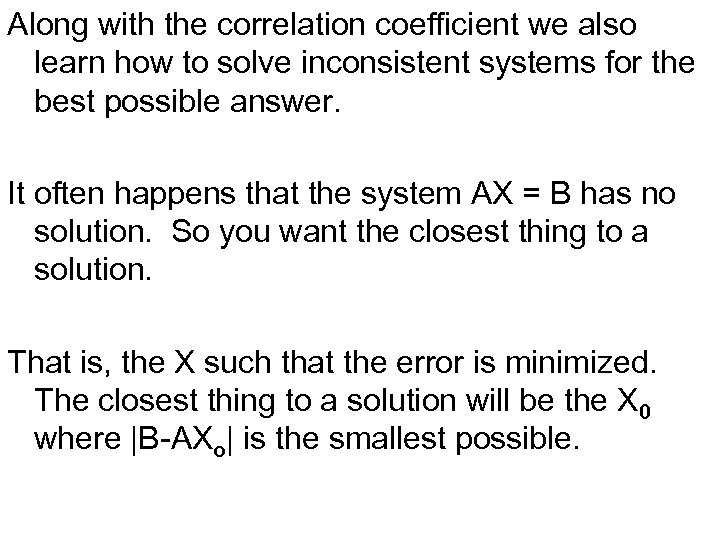Along with the correlation coefficient we also learn how to solve inconsistent systems for the best possible answer. It often happens that the system AX = B has no solution. So you want the closest thing to a solution. That is, the X such that the error is minimized. The closest thing to a solution will be the X 0 where |B-AXo| is the smallest possible.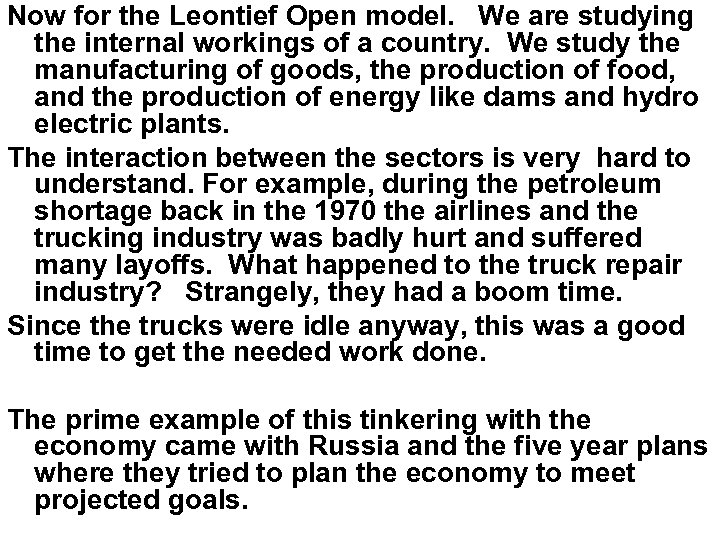Now for the Leontief Open model. We are studying the internal workings of a country. We study the manufacturing of goods, the production of food, and the production of energy like dams and hydro electric plants. The interaction between the sectors is very hard to understand. For example, during the petroleum shortage back in the 1970 the airlines and the trucking industry was badly hurt and suffered many layoffs. What happened to the truck repair industry? Strangely, they had a boom time. Since the trucks were idle anyway, this was a good time to get the needed work done. The prime example of this tinkering with the economy came with Russia and the five year plans where they tried to plan the economy to meet projected goals.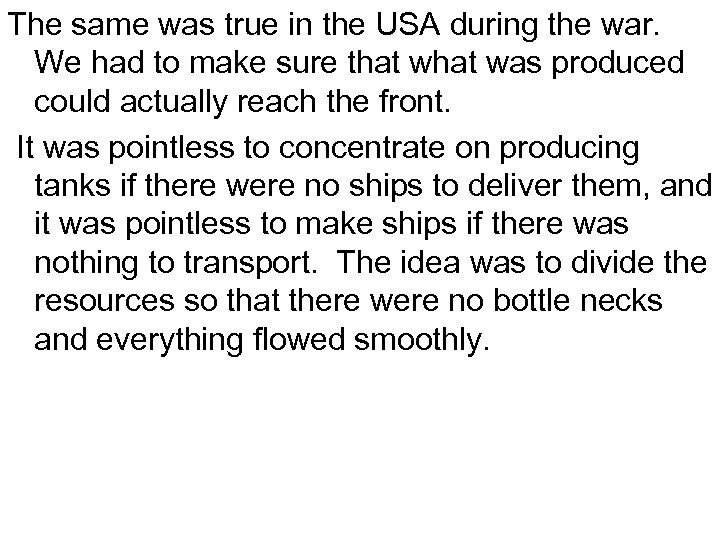The same was true in the USA during the war. We had to make sure that was produced could actually reach the front. It was pointless to concentrate on producing tanks if there were no ships to deliver them, and it was pointless to make ships if there was nothing to transport. The idea was to divide the resources so that there were no bottle necks and everything flowed smoothly.Now days, one can use the idea to work the stock market. When you read in the paper that there is a new oil embargo, or that there is a ban on DDT, or what ever, the big boys have already had that information and the stocks market prices have already been adjusted. What is left is for one to get in on the second tier. Those are the industries which are not so obviously affected.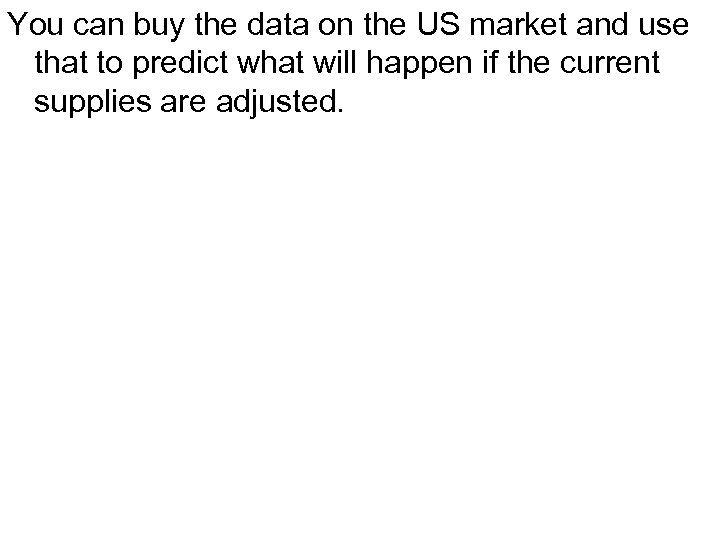You can buy the data on the US market and use that to predict what will happen if the current supplies are adjusted.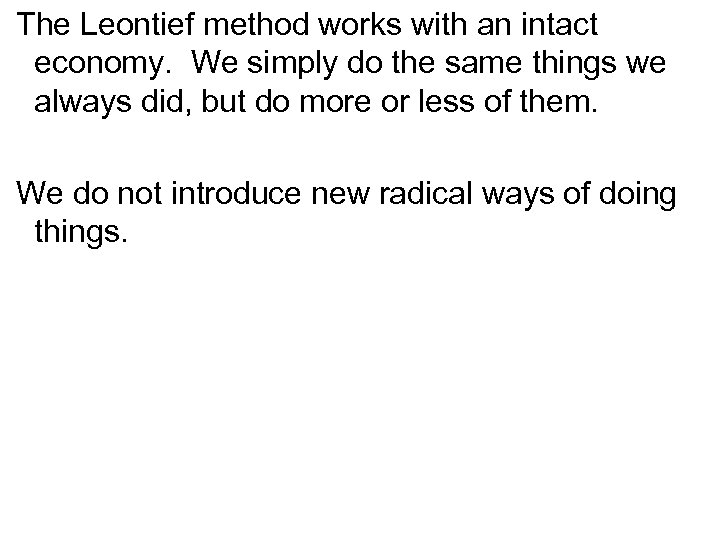The Leontief method works with an intact economy. We simply do the same things we always did, but do more or less of them. We do not introduce new radical ways of doing things.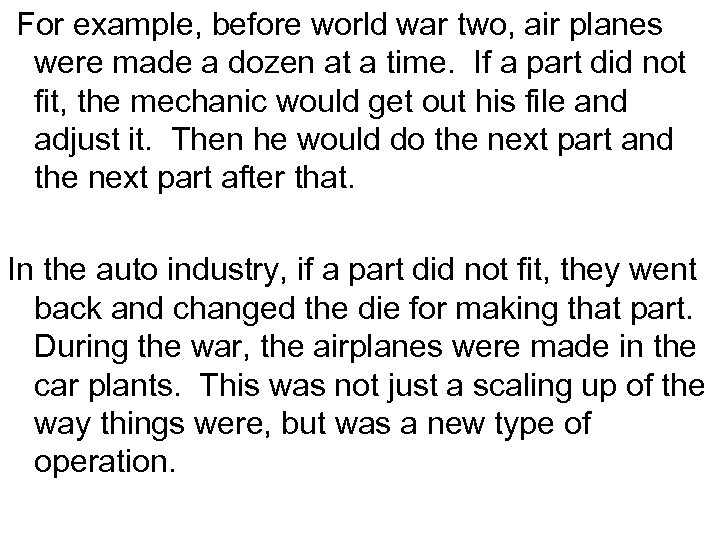For example, before world war two, air planes were made a dozen at a time. If a part did not fit, the mechanic would get out his file and adjust it. Then he would do the next part and the next part after that. In the auto industry, if a part did not fit, they went back and changed the die for making that part. During the war, the airplanes were made in the car plants. This was not just a scaling up of the way things were, but was a new type of operation.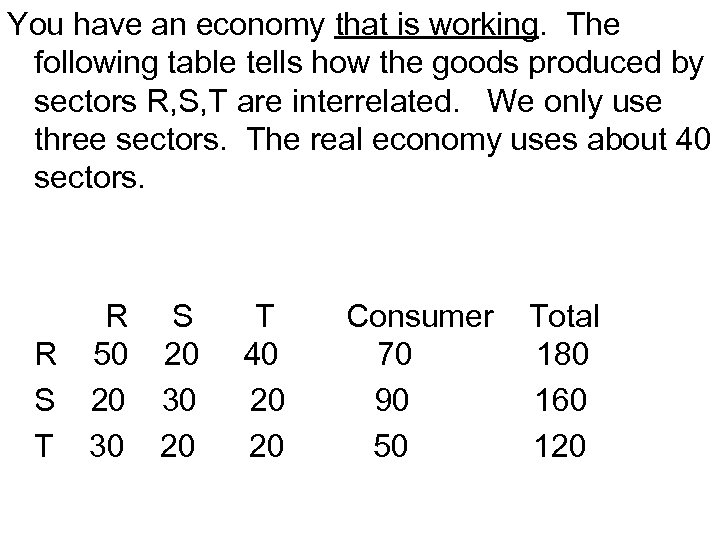You have an economy that is working. The following table tells how the goods produced by sectors R, S, T are interrelated. We only use three sectors. The real economy uses about 40 sectors. R S R 50 20 S 20 30 T 30 20 T 40 20 20 Consumer 70 90 50 Total 180 160 120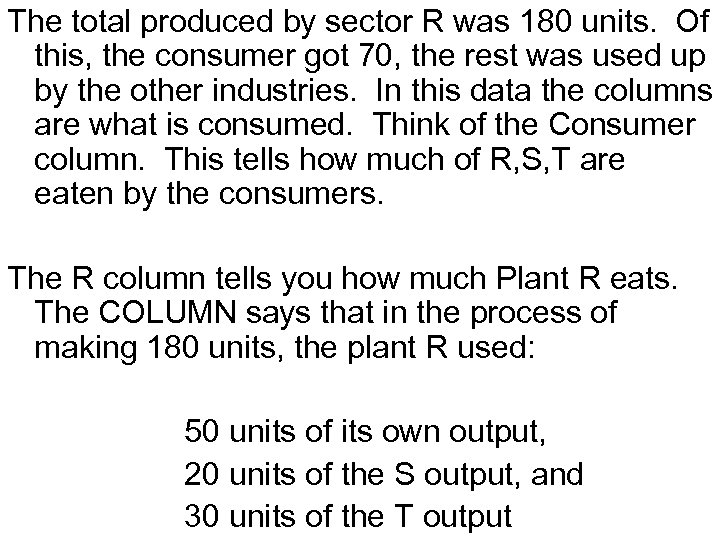The total produced by sector R was 180 units. Of this, the consumer got 70, the rest was used up by the other industries. In this data the columns are what is consumed. Think of the Consumer column. This tells how much of R, S, T are eaten by the consumers. The R column tells you how much Plant R eats. The COLUMN says that in the process of making 180 units, the plant R used: 50 units of its own output, 20 units of the S output, and 30 units of the T output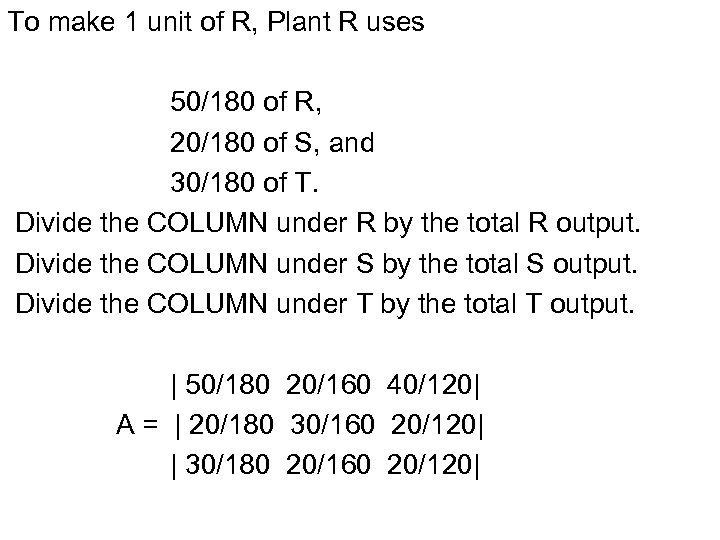To make 1 unit of R, Plant R uses 50/180 of R, 20/180 of S, and 30/180 of T. Divide the COLUMN under R by the total R output. Divide the COLUMN under S by the total S output. Divide the COLUMN under T by the total T output. | 50/180 20/160 40/120| A = | 20/180 30/160 20/120| | 30/180 20/160 20/120|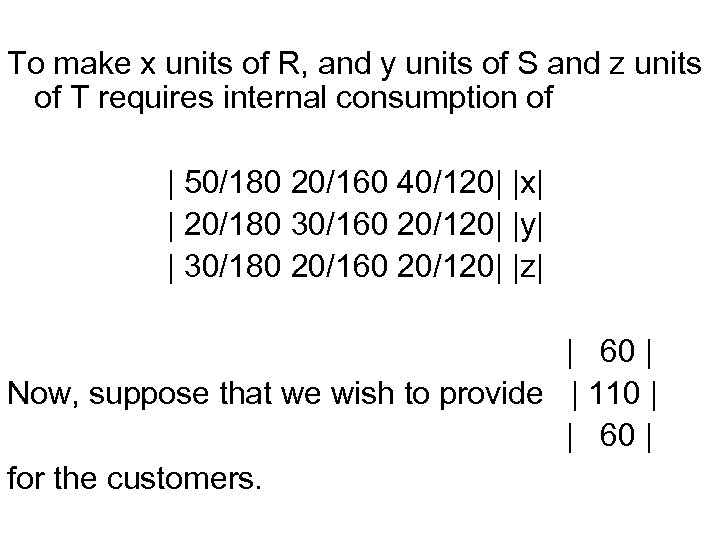To make x units of R, and y units of S and z units of T requires internal consumption of | 50/180 20/160 40/120| |x| | 20/180 30/160 20/120| |y| | 30/180 20/160 20/120| |z| | 60 | Now, suppose that we wish to provide | 110 | | 60 | for the customers.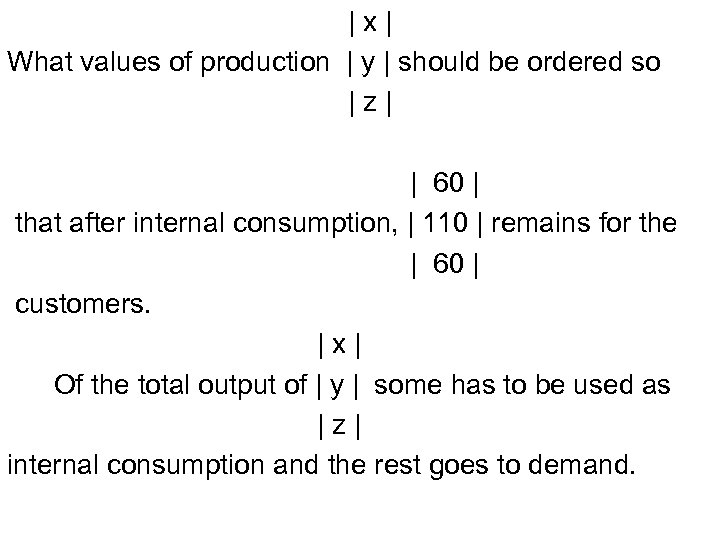|x| What values of production | y | should be ordered so |z| | 60 | that after internal consumption, | 110 | remains for the | 60 | customers. |x| Of the total output of | y | some has to be used as |z| internal consumption and the rest goes to demand.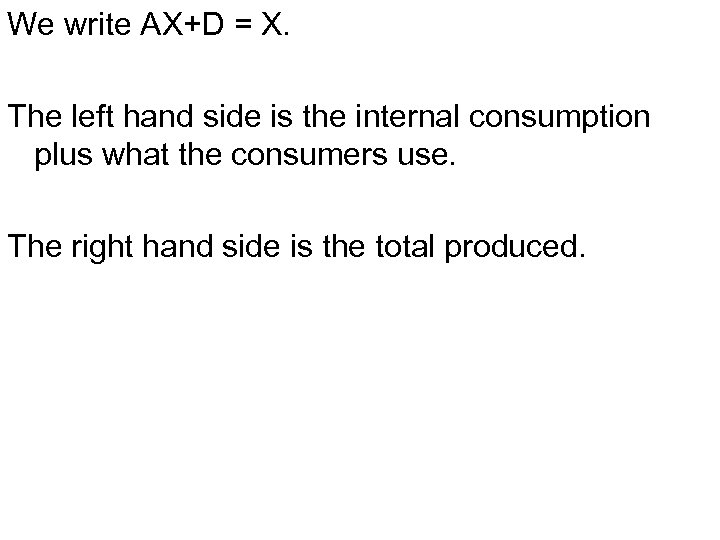We write AX+D = X. The left hand side is the internal consumption plus what the consumers use. The right hand side is the total produced.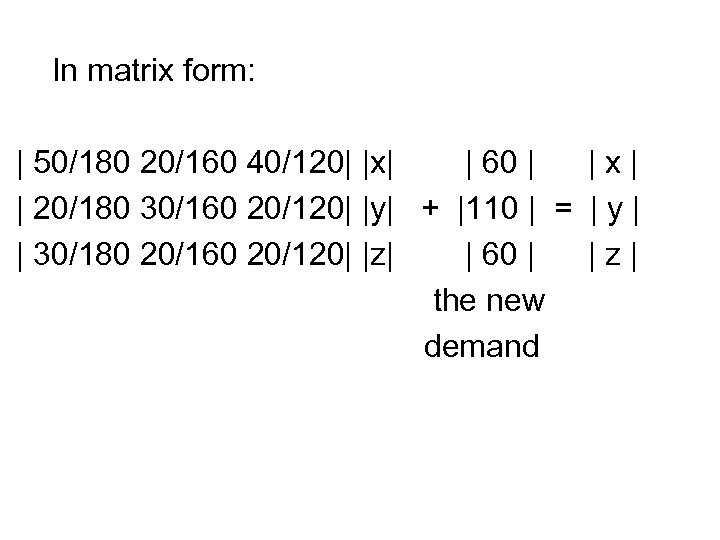In matrix form: | 50/180 20/160 40/120| |x| | 60 | |x| | 20/180 30/160 20/120| |y| + |110 | = | y | | 30/180 20/160 20/120| |z| | 60 | |z| the new demand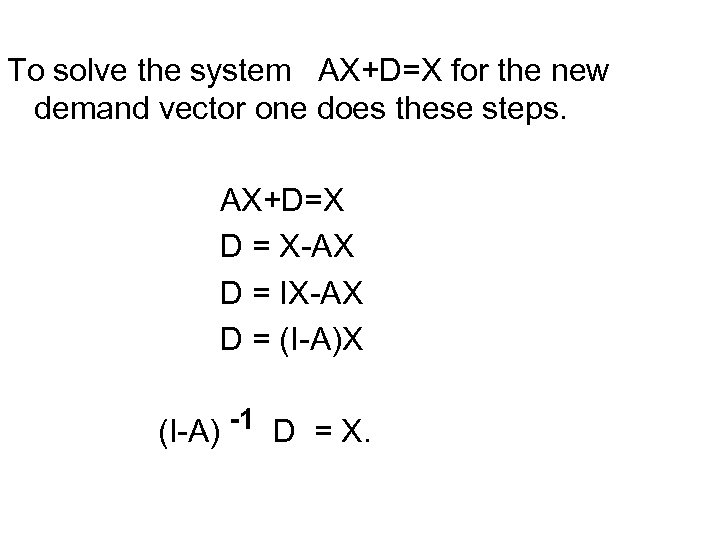To solve the system AX+D=X for the new demand vector one does these steps. AX+D=X D = X-AX D = IX-AX D = (I-A)X (I-A) -1 D = X.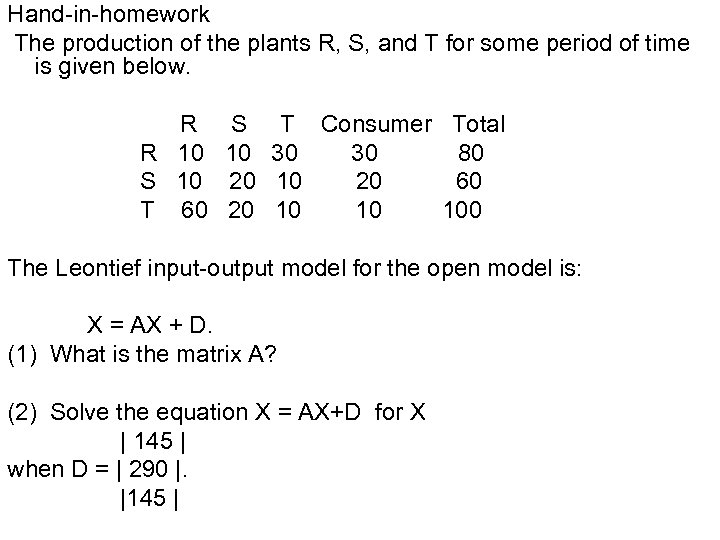Hand-in-homework The production of the plants R, S, and T for some period of time is given below. R R 10 S 10 T 60 S 10 20 20 T Consumer Total 30 30 80 10 20 60 10 10 100 The Leontief input-output model for the open model is: X = AX + D. (1) What is the matrix A? (2) Solve the equation X = AX+D for X | 145 | when D = | 290 |. |145 |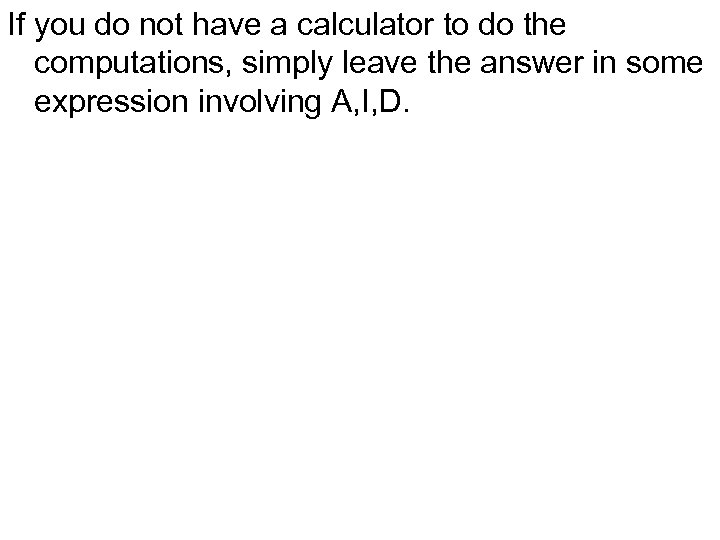If you do not have a calculator to do the computations, simply leave the answer in some expression involving A, I, D.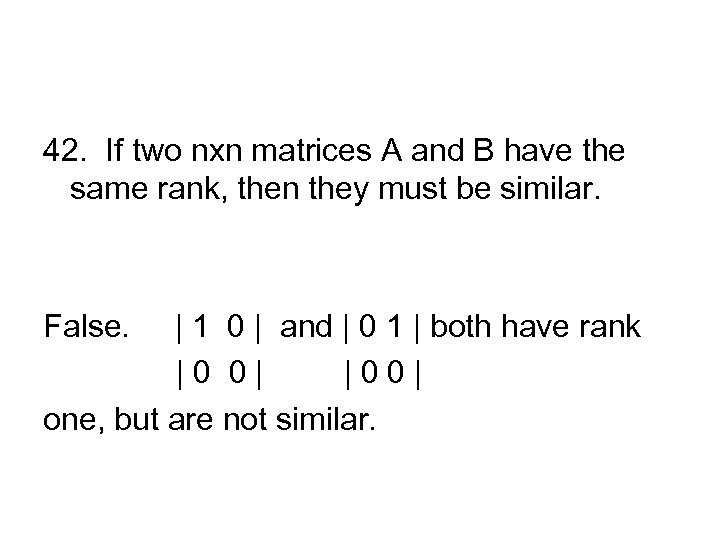42. If two nxn matrices A and B have the same rank, then they must be similar. False. | 1 0 | and | 0 1 | both have rank |0 0| |00| one, but are not similar.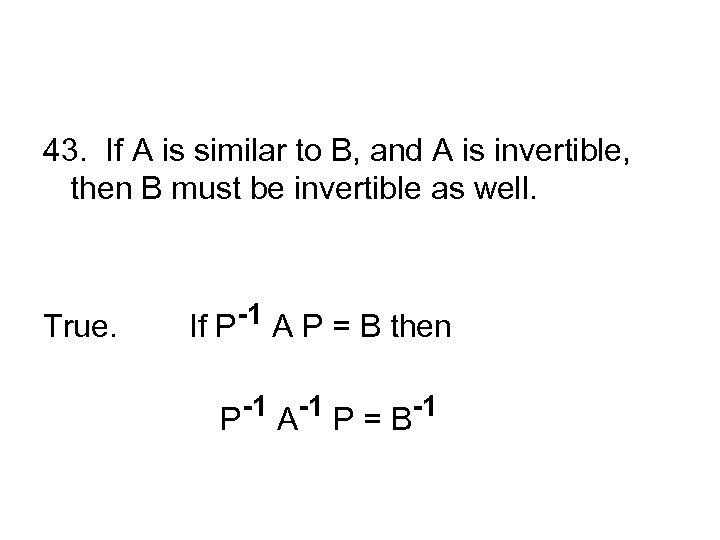43. If A is similar to B, and A is invertible, then B must be invertible as well. True. If P-1 A P = B then P-1 A-1 P = B-1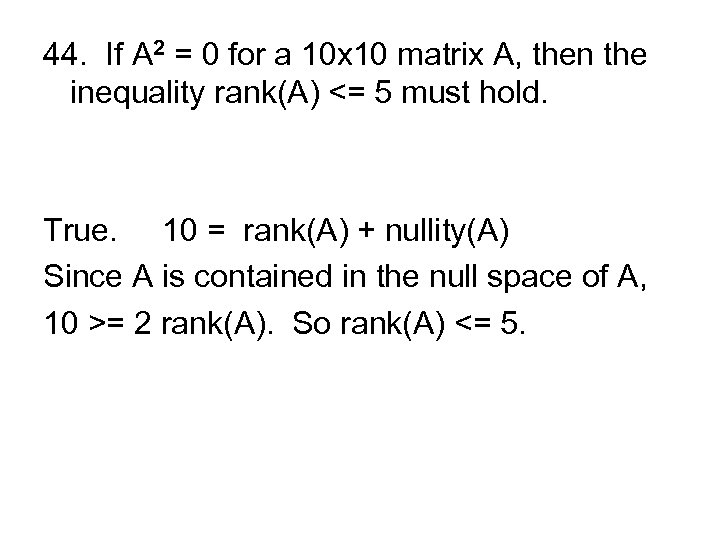44. If A 2 = 0 for a 10 x 10 matrix A, then the inequality rank(A) <= 5 must hold. True. 10 = rank(A) + nullity(A) Since A is contained in the null space of A, 10 >= 2 rank(A). So rank(A) <= 5.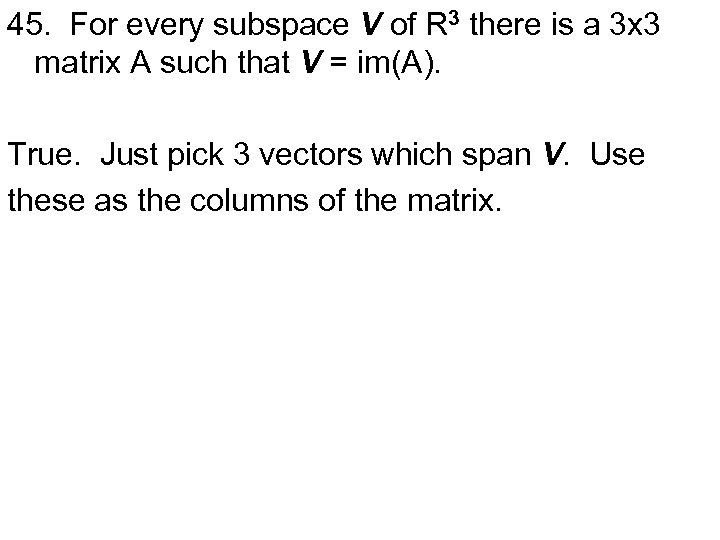45. For every subspace V of R 3 there is a 3 x 3 matrix A such that V = im(A). True. Just pick 3 vectors which span V. Use these as the columns of the matrix.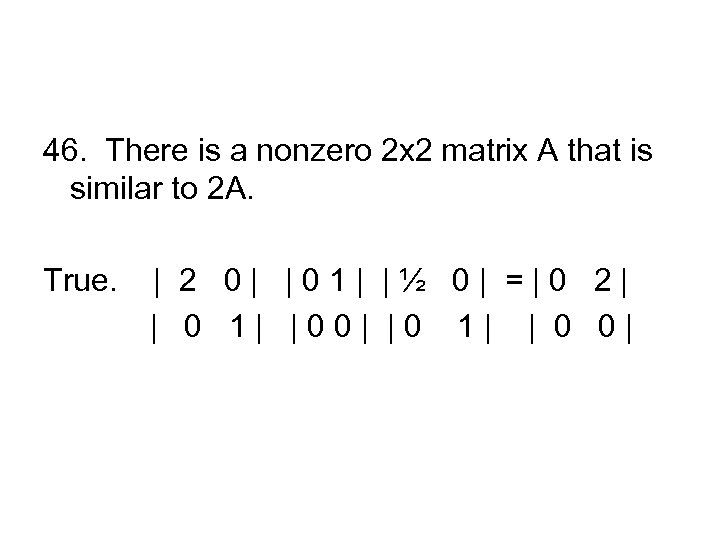46. There is a nonzero 2 x 2 matrix A that is similar to 2 A. True. | 2 0| |01| |½ 0| =|0 2| | 0 1| |00| |0 1| | 0 0|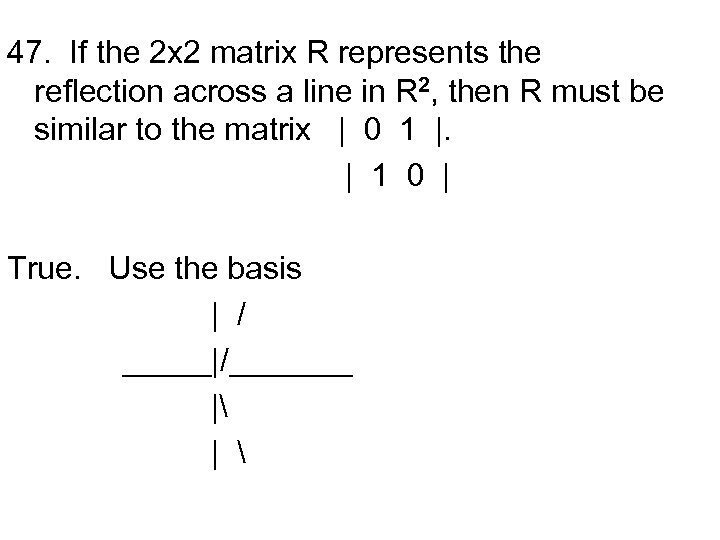47. If the 2 x 2 matrix R represents the reflection across a line in R 2, then R must be similar to the matrix | 0 1 |. | 1 0 | True. Use the basis | / _____|/_______ | |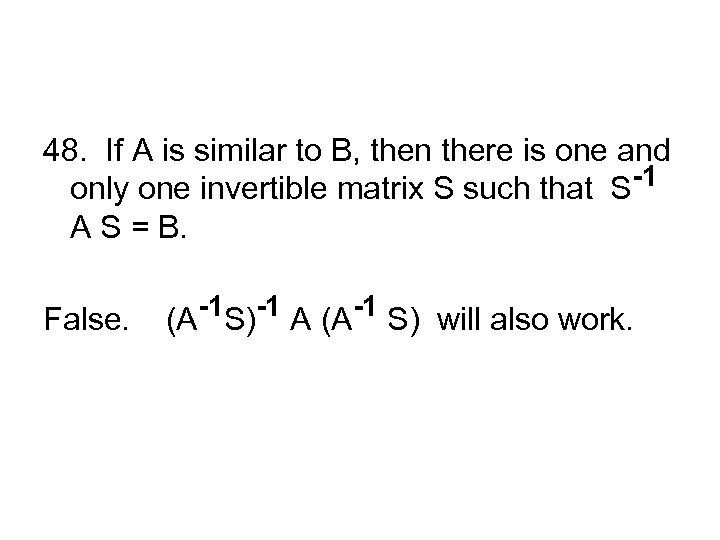48. If A is similar to B, then there is one and only one invertible matrix S such that S-1 A S = B. False. (A-1 S)-1 A (A-1 S) will also work.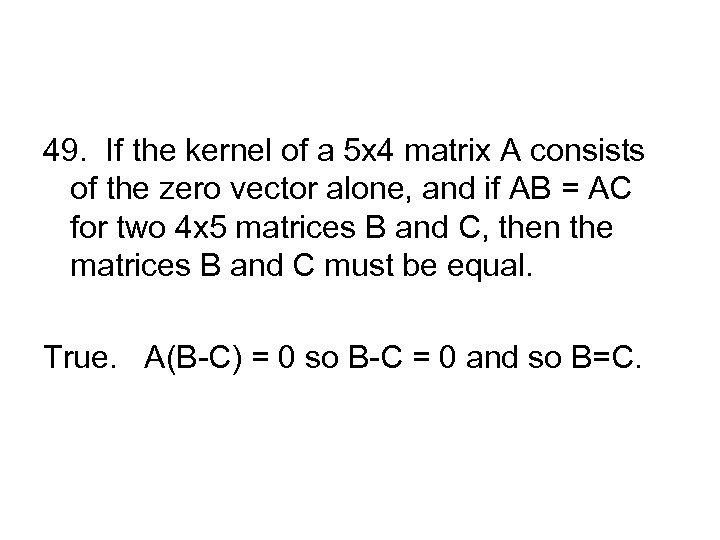49. If the kernel of a 5 x 4 matrix A consists of the zero vector alone, and if AB = AC for two 4 x 5 matrices B and C, then the matrices B and C must be equal. True. A(B-C) = 0 so B-C = 0 and so B=C.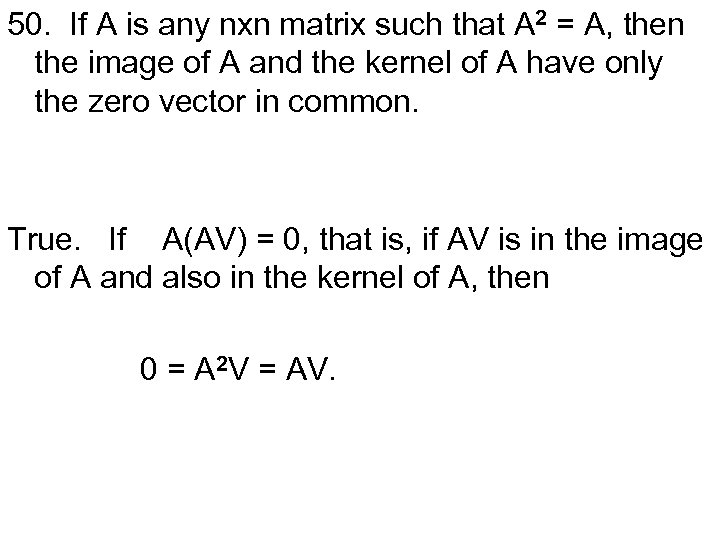50. If A is any nxn matrix such that A 2 = A, then the image of A and the kernel of A have only the zero vector in common. True. If A(AV) = 0, that is, if AV is in the image of A and also in the kernel of A, then 0 = A 2 V = AV.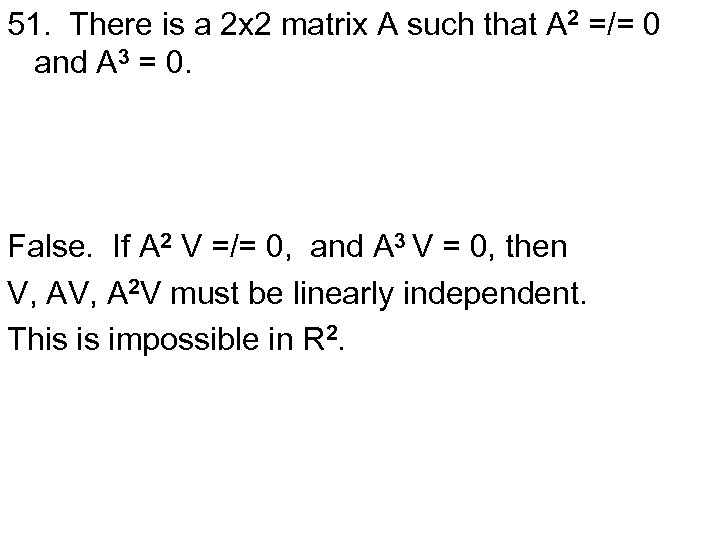51. There is a 2 x 2 matrix A such that A 2 =/= 0 and A 3 = 0. False. If A 2 V =/= 0, and A 3 V = 0, then V, A 2 V must be linearly independent. This is impossible in R 2.